The Procedure of
Conceptual Integration
Introduction

During more than 25 centuries western thinking was dominated by the dualistic paradigm described by Aristotle's syllogism. A statement is true or false, exclusively one of both, and all of intellectual activity consists of evaluating, arguing or refuting the statement. This approach got a boost during Renaissance, where "cartesian" deductive logical and mathematical thinking was considered as the absolute requisite for any kind of scientific, "experimental", reliable thinking.

Apart from its contributions to deductive thinking, this approach is misleading in at least three of its explicitly or implicitly suggested conclusions:

(1) there is no category between true and false;
(2) science and technology are making progress thanks to logical deductions and experimental procedures;
(3) "sciences" which so far are unable to apply the exact, experimental procedure can't be rated as scientifically reliable, and remain in the realm of mythical, obscure, fictitious and unreliable thinking.
During the second part of the 20th century, after the umpteenth deception about the blessings of scientific progress, tentatives towards a more holistic, non-aristotelic approach of reality were launched. This trend is masterly pictured by authors as Fritjof Capra. Many approaches, from postmodernism to New Age, recommend a less dualistic, mutually-exclusive way of thinking. But up to now a central paradigm was not clearly defined. In this essay, integration is proposed as such a paradigm.

Definition

As defined elsewhere, integration can be defined as 

a process of combination of elements that, at first approach, seem to be incompatible or even conflictuous, but, after a bit of analysis and re-synthesis (leading to reformulation or re-orientation), prove to be rather complementary.

Symbolically this process could be represented by:

(A,B) Æ [A>>A'] & [B>>B'] Æ {A'B'}

where A en B are elements in their primary, "unintegrable" state, that are "retroduced" to their "essential core" [AÆA'] & [BÆB'], what makes them "integrable", and then combined into a new unity {A'B'}. Æ here means "to provoke a certain fact or transformation". and >>here means "to transform into". We use the three kinds of brackets in a different sense: (collection of elements), [process], {integration}.

We would like to introduce the function of Y (Psi) meaning "to be reduced to its essential core", so that:

Y(A) = A'

and v  as the inverse function, the "eduction"

A = v(A')

so that A is an eduction of A', meaning that we can come from A' to A by making A' less general.

Likewise we propose to introduce the fundamental function W (Omega) to describe the integrative process in short:

W (A,B)= {A'B'}

To make the indication "a more integrative version" more precise than A' relative to A (A' meaning just "vaguely related to") we propose to introduce the index # meaning "more integrative".

Symbolically, the above statements can be written as

(A,B) Æ [A>>A#] & [B>>B#]Æ {A#B#}

Y(A) = A#

A = v (A#)

W (A,B) = {A#B#}

Assumptions

The paradigm of integration is based upon some assumptions, some of them conflictuous with the fundamental logical postulates.

1. The probability that a theory is completely true, is practically nill

The probability that a theory, an insight, a concept, a hypothesis, an idea, etc. is completed, completely exact and true, is practically inexistent. Each idea, etc. will probably be enhanced some day. So between "true" and "false" a third category has to be intercalated, being defined as "theories that are far more better than previous theories, but not as perfect as the final theory".

The classical, dualistic, aristotelian-cartesian categories: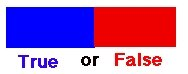In the integrative approach we discern three categories: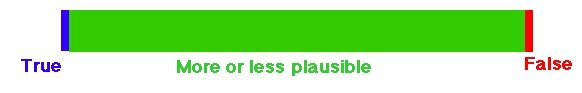In practice, we could probably put all existing theories, concepts, etc., in the middle category, making both classical logical categories obsolete, i.e. only existing as a theoretical concept, unless we're working with extremely simple data, e.g. natural numbers and other concentional concepts.

As the "plausibility" category is very extended, it seems useful to introduce a measure of plausibility (P). This measure can only be used in a relative sense, i.e. by comparing two or more theories.

If we define TE as the Theories of Einstein, and TN as the Theories of Newton, we can state that:

P(TE) > P (TN)

Or:

Y(TN) = TE

TN = v (TE)

As explained in the General Systems Theory, and Logics knowledge of any rather complex system presents a progressive development: as with a spiral, each new experience or intellectual confrontation may lead to a more comprehensive conceptualization.

E1(A) Æ a1

a1 + E2(A) Æ a2

a2 + E3(A) Æ a3

generally described as

an-1 + En(A) Æ an

A is a phenomenon, a part of reality to be conceptualized. E is an experience and/or an intellectual communication. E(A) is an experience with phenomenon A , and E1(A), E2(A)... are consecutive experiences. a1, a2, ..., anare consecutive visions, hypotheses about phenomenon A , becoming progressively more integrative. A graphical time stages scheme could also represent this procedure: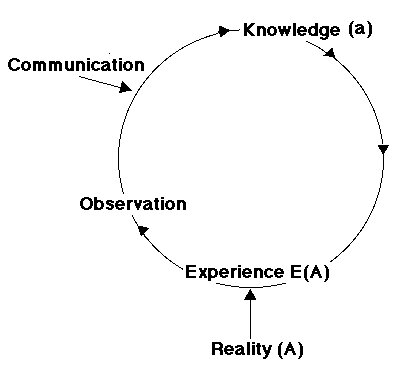This means that at each given moment, each concept may become more complete, more realistic, more comprehensive, more integrative.

The historically most significant example is the theory of Newton, enhanced by Einstein. This example is particularly striking because during centuries the theories of Newton were quoted as the illustration par excellence of scientific, aristotelic-cartesian thinking. Let's take Newton's Law of the Addition of Speeds:Einstein proposed an elaborated formula: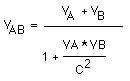This means that at relatively slow speeds (v << c), the denominator practically equals 1, so we find back Newton's formula. But at high speeds the denominator increases making the total speed significantly lower than Newton predicted, and limiting even the maximal speed at the speed of light, c. Newton's formula appears to be a reduction a simplifictaion of Einstein's elaborated formula, but at "normal" speeds on earth it was impossible to discover the inaccuracy of Newton's "simple" formula.

2. The 'complements' operator - the non-existence of contradictions

As there exists more than one category of exactness, the opposition and mutual exclusion of two statements (about the same phenomenon) that are not similar or reduceable to each other, disappear. Besides the fundamental categories equals (=) and is unequal (<>) we should like to introduce a third, much more useful operator: complements (¿)

Where in the traditional approach statements as

psychoanalysis <> behaviourTheory
science <> religion
theoryOfNewton <> unexplainableEvidence

seem very justified, in an integrative approach they are... false, because:

psychoanalysis ¿ behaviourTheory
science ¿ religion
theoryOfNewton ¿ unexplainableEvidence

Hence:

W (psychoanalysis, behaviourTheory, ...) = integrativePsychology
W (science, religion, ... ) = hyperphysics
W (theoryOfNewton, unexplainableEvidence ) = theoryOfEinstein

("Hyperphysics" being the name that Teilhard and Wildiers used as the scientific label for their evolutionary, cosmic and integrative approach).

This second postulate can be formulated another way: the probability that in two statements, which seem mutually exclusive of paradoxical, one is true and the other is false, much lower than the probibility that both pseudo-conflicting statements are complementary, i.e. true in certain circumstances. Practically speaking: all so called contradictions are most probably complements.

3. An integrative theory is more plausible than its non-integrative elements

The degree of plausibility increases with integration, at least if no logical errors are made. This can be explained by the fact that the plausibility of the integrative view is at least as high of the plausibility of the most plausible of its complementary elements.

So we can state that

P(A#) > P(A)

and, more in detail, if:

W (A1,  A2 ,  A3 , ...) = A#
then:
P(A#) > P(A1)
P(A#) > P(A2)
P(A#) > P(A3)
...

This has a very important consequence: to achieve the highest possible plausibility, one has to integrate. Or, otherwise stated, the Plausibility is the highest with theories that take into account the greatest number of phenomena, the greatest number of arguments.

Graphically represented:A "proof" based upon the integration paradigm can be made by arguing, mathematically or logically, that the former, non-integrative theories can be reached by an eduction (v) of the integrative theory.

If

W (A1,  A2 ,  A3 , ...) = A#

then the maximal plausibility for  A# (replacing the obsolete concepts true and exact) is reached when

A1 = v (A#)
A2 = v (A#)
A3 = v (A#)

i.e. if we can (d)educe each elementary theory from the integrative, by restricting the conditions of applicability or observation. This is a proof, in the scientific meaning of the word, that the integrative theory is (more) true (preferably: plausible) than the non-integrative ones.

This edutive operation cabn easily be done with Einstein's theory about the addition of velocity, that mathematically can reduced to Newton's formula by putting v as extremely low, so that the denominator practically equals 1, and can be removed in the formula.

This third assumnption has many important implications for science in general, especially for these sciences that are seemingly (still) inaccessible for the exact-scientific method. These consequences apply as well to the curriculum of the studies, as to the structure of scientific papers. In another article we will extend on that topic.

The integrative procedure

1. Introduction

Deduction and induction. The process of integration, leading to the formulation of a new, more plausible hypothesis, is part of the indcution process, taht is still unconscious and inaccessible for conscious reasoning. All the Principles of Logic, from Socrates to Descartes and Leibniz, in fact are Principles of Deductive Logic. An Inductive Logic is still to be elaborated. This integrative process can be seen as the kernel of such a paradigm.

In the next scheme the process of thinking is depicted. In intelligent beings, Reality is, at least in part, observed, and out from these observations some general tendencies are abstracted. These are the hyotheses, the knowledge, general rules and laws. The hypothesis formulation process is called induction. From these abstract hypotheses, conclusions can be drawn by logical, deductive thinking. Generally this operation consists in replacing abstract data by concrete numbers, and performing some mathematical transformations. Science is a collection of conscious rules, i.e. operationalized and measurable. Also in exact science, the elaboration of a scientific hypothesis is an intuitive process. In fact, science only controls the "exactness" of the intuitively formulated hypotheses.

In many cases, the hypothesis itself remains hidden in the realm of the unconscious, and is then called intuition. In this case also the deductive process is largely uncuncious and intuitive, e.g. art, musical improvisation.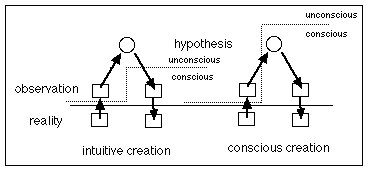The integration process is the elaboration of a new, more general hypothesis out of some particular hypotheses.

Because it is still largely unconscious, this process can not ye be described in detail, not yet operationalized, and not yet programmable for a computer. All computers are still deductive up to now, although when the possibilities are limited, a pseudo-inductive routine can be elaborated, like in computer chess programs: a mathematical calculation of all possible reactions is made, and a quick statistical evaluation is made of the outcome of each possibility, and then a mathematically guided choice is automatically made.

But although we can't yet describe the process in detail, we can identify several subphases, making the theoretical challenge more realistic, and perhaps coming closer to the long expected discovery of an Inductive Logic. We also will offer some auxiliary tools to enhance this subconscious integrative process.

2. Eduction (v)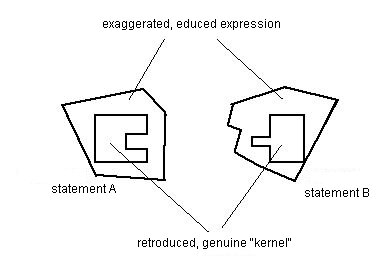Let's consider two statements, A en B. The seem irreconcilable:

A <> B
But let's suppose that, most probably, both observers were sufficiently intelligent not to be completely wrong, but were observing different situations: different factors elicited different behaviours in the same object. So trhe different perceptions of the same object are in fact compatible, save for the exaggerate generalization (eduction), unconsciously made in the absence of correcting phenomena, thus making the statements unnecessarily incompatible.

3. Retroduction (Y)

The solution is that both observers retroduce their statements, by adding nuances or limiting conditions, making an integration possible.

We postulate that A ¿ B, and we retroduce A and B to A# and B# by

Y(A) = Aand  Y(B) = B#

4. Combination (+)

At this point an integration, now nothing more than a simple combination,  will be possible:

W (A, B) =  {A#B#}To perform this integration, several methods seem useful:

- Each observer can be placed in the situation of the other observer, and discover that his stement needs some more nuance.
- The observers can communicate their different approach to each other, believe each other, and by empathy understand the limits of their own experience, and make together an integrative hypothesis satisfying both of them.
- One observer can accept the apparent contradictory statements as complementary, and try to formulate an integration.
5. Auxiliary methods

The intuitive integration probably will be inspired by analogies, and especially by analogies with fundamental features of reality described by the General Systems Theory, and assisted by inductive Logics.

In another article, several auxiliary tools to enhance intuitive integration, are described.

 Roose, K., 1985, Het geheim van het geluk, Kluwer, Antwerpen-Deventer